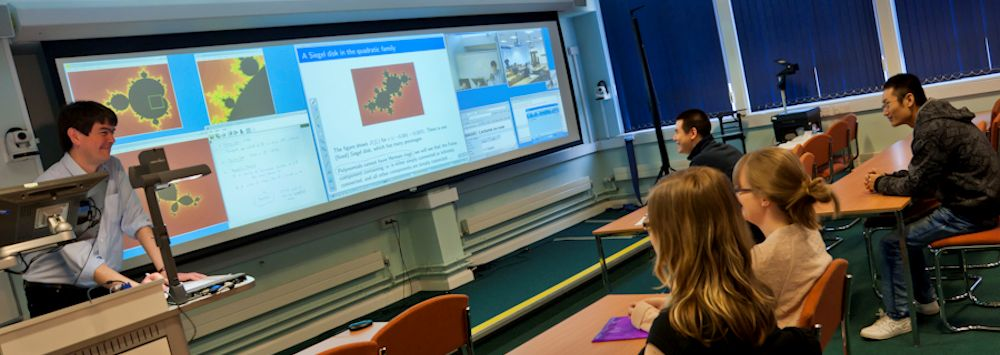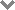# Stochastics Seminars

• ### Friday 25th October 2019 - Fatou's Lemmas for Varying Probabilities and their Applications to Sequential Decision MakingStochastics Seminar

Speaker: Distinguished Professor Eugene A. Feinberg, Stony Brook University (USA)

16:00 MATH-211

Abstract: The classic Fatou lemma states that the lower limit of expectations is greater or equal than the expectation of the lower limit for a sequence of nonnegative random variables. This talk describes several generalizations of this fact including generalizations to converging sequences of probability measures. The three types of convergence of probability measures are considered in this talk: weak convergence, setwise convergence, and convergence in total variation. The talk also describes the Uniform Fatou Lemma (UFL) for sequences of probabilities converging in total variation. The UFL states the necessary and sufficient conditions for the validity of the stronger inequality than the inequality in Fatou's lemma.

We shall also discuss applications of these results to sequential optimization problems with completely and partially observable state spaces. In particular, the UFL is useful for proving weak continuity of transition probabilities for posterior state distributions of stochastic sequences with incomplete state observations known under the name of Partially Observable Markov Decision Processes. These transition probabilities are implicitly defined by Bayes' formula, and general method for proving their continuity properties have not been available for long time.

This talk is based on joint papers with Pavlo Kasyanov, Yan Liang, Nina Zadoianchuk, and Michael Zgurovsky.

• ### Wednesday 12th June 2019 - Comparison Theorem of Stochastic Differential EquationsSpeaker: Chenggui Yuan (Swansea University)

16:00 MATH-117

Abstract: In this talk, the existence and uniqueness of strong solutions to distribution dependent neutral SFDEs are proved. We give the conditions such that  the order preservation of  these equations holds.  Moreover, we show these conditions are also necessary when the coefficients are continuous. Under sufficient conditions, the result extends the one in the distribution independent case, and the necessity of these conditions  is new even in distribution independent case.

• ### Friday 1st March 2019 - Stochastic Porous Media Equations: An Entropy ApproachSpeaker: Konstantinos Dareiotis (Max Planck Institute for Mathematics in the Sciences, Leipzig, Germany)

12:00 MATH-105

Abstract: Nonlinear diffusion equations describe macroscopic phenomena such as flow of gas or fluid in a porous medium, heat propagation with temperature-dependent conductivity, and the evolution of crowd-avoiding populations. Often, a source term is present that forces/removes mass into/from the system. This source term can be random. As a particular example, generalised stochastic porous media equations (SPME) appear as scaling limits of the empirical measure of interacting branching diffusion particle systems. The interaction leads to a non-linear, degenerate second order operator in the drift, while the randomness of the branching mechanism leads to a non-linear noisy source term. We will discuss the main difficulties towards well posedness of these equations and introduce the concept of entropy solutions. In the class of entropy solutions we obtain well-posedness, L 1 -contraction, and stability estimates. Our results cover the full range of powers of the porous medium operator and allow for nonlinearities in the noise that are 1/2-Hölder continuous. This is a joint work with Mát´e Gerencs´er and Benjamin Gess.